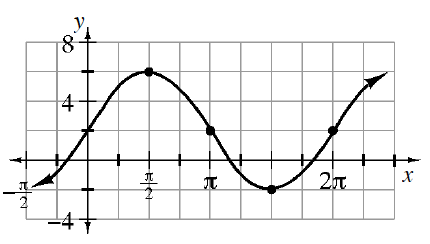### Home > INT3 > Chapter 10 > Lesson 10.1.4 > Problem10-58

10-58.

Write a possible equation for the graph below.Determine how the graph is altered from the graph of $y = \sin\left(x\right)$. What is the amplitude? Where is the midline?

$y = 2 + 4 · \sin\left(x\right)$